Select Page

# Hamming Code For Error Correction

When sender sends data to receiver then errors may occur. To detect and correct these errors, error correction codes are used.

There are two methods to handle the error Correction

1. Backward error correction: After error detection , retransmission of entire data unit is called backward error correction.

2. Forward error correction: In forward correction, after error detection, the receiver uses the error-correcting code which corrects the errors automatically.

Hamming Code is the best method which uses the forward error correction technique to correct the data. It was developed by R.W Hamming.

## Hamming code

According to hamming code, some redundant bits are added with actual data bits and transmitted. At receiver side these bits are checked, if any there exist any bit error then it is also corrected.

In Hamming code, Redundancy bits are not fixed. If data bits are increased then redundancy bits are also increased. At the time of sending data, we don’t know the number of redundancy bits.

There are various steps to understand hamming code.

### Step 1: No. of Redundancy Bits “r”

Consider “r” is the number of redundant-bits and “d” is the total number of the data-bits. The number of redundant bits r can be calculated by using the formula:

2r>=d+r+1

Keep in Mind: consider the smallest value of “r” which satisfy the above formula.

#### Understand With Example

Consider the data (1010) which needs to be sent.

• Total number of data bits “d” = 4
• Number of redundant bits “r” will be 2r >= 4+r+1

Hence, the value of “r” is 3 because 3 is the smallest value of “r” that satisfies the above equation.

• Total number of bits (data + redundancy) = d+r = 4+3 = 7;

### Step 2: Position for each Redundancy bits “r”

Position of redundancy bit will be calculated through the formula of 2n. If there are three redundancy bits r1, r2 and r3 then their corresponding positions are 1, 21, and 22.

• First position of redundancy bit (r1) = 20= 1
• Second position of  redundancy bit (r2) = 21= 2
• Third position of redundancy bit (r3)  = 22= 4   and so on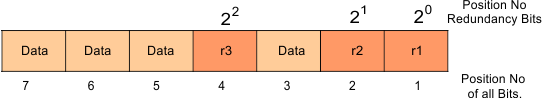As we have 4 data bits (1010) put them in their positions as below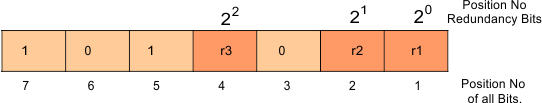### Step 3: Determining The Value Of Each Redundancy bits “r”

Each redundancy bits holds its fix position data bits always. As given below

• r1 fix positions of data bits are all odd positions as 1,3,5,7 and so on.
• r2 fix positions of data bits are 2,3 and then skip 4,5 and then consider 6,7.
• r3 fix positions of data bits are 4,5,6,7 and then 4-skip (8,9,10,11) and then 4 consider and so on.

For the purpose of even parity, Count the number of 1’s for each r1,r2 and r3 through its fix positions. If the number of 1’s are even then that redundancy bit (r) will be 0 otherwise 1.

So value of r1,r2 and r3 is given below

• r1 Value = all odd positions as 1,3,5,7 = 011 = 0
• r2 Value = positions of 2,3,6,7 = 011 = 1
• r3 Value = position of 4,5,6,7 =  101 = 0

### Step 4: Put The Values Of Redundancy Bits To Their Positions

There are 4 data bits (1010) and 3 redundancy bits (010) as given under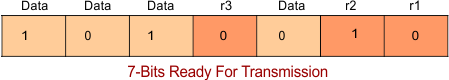Suppose the 6th bit is changed from 0 to 1 at the receiving end as given below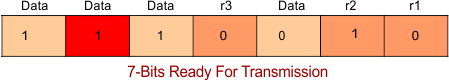Now again calculate the redundancy value for r1,r2,3  to correct the error.

• r1 Value = all odd positions as 1,3,5,7 = 1100 = 0
• r2 Value = positions of 2,3,6,7 = 1101 = 1
• r3 Value = position of 4,5,6,7 =  1110 = 1

The binary representation of redundant bits, i.e. r3r2r1 is 110, and its corresponding decimal value is 6. Therefore, the error occurs in a 6th bit position. The data-bit value must be changed from 1 to 0 to correct the error.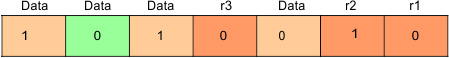Help Other’s By Sharing…